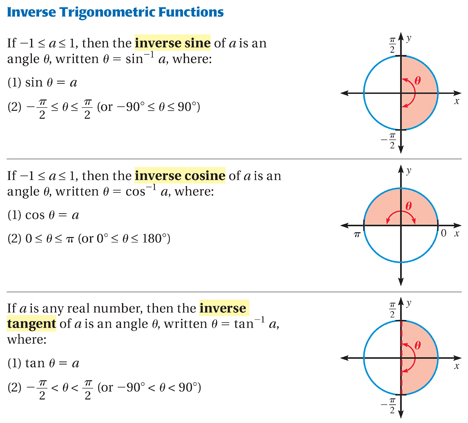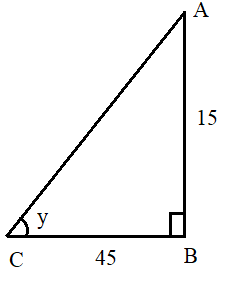#### Arctangent Calculator

Result:

 Angle in degrees: 0 Angle in radian: 0

### What is Arctangent?

Arctangent (or arctan) is the inverse of the tan function. In a right-angled triangle, the tan of an angle y is defined as the ratio of the side opposite to the angle, to the adjacent side. When we take arctan of this ratio, we get the measure of the angle y.

Consider the right-angled triangle below.In this triangle, the right angle is at B. Tan of the angle y is given by

$$tan\, y\, =\, {opposite \over adjacent} \,=\,{AB\over BC} = x$$

To find the angle y when the ratio AB/BC is known, we use the following formula for arctan.

$$y\, =\, tan^{-1}{opposite \over adjacent} \,= tan^{-1}\,{AB \over BC}\, = tan^{-1} x$$

The expression y = tan-1⁡x, means that tan y = x , when -π/2 < y < π/2.

Arctan(x)=y is defined as the set of all angles whose tan is x. It should be noted here that the -1 is not an exponent and hence, tan-1⁡x ≠ 1 / tan x .

### Properties of the arctan function

Given below are some properties of the arctan function.

Angle – The angle is the parameter which is calculated when tan inverse (or arctan) of the angle is known.

Domain – The arctan of the parameter whose tan is x. This value can be any real number.

Range – The angles for which we can find tan^(-1)⁡x. This value is -π/2 < y < π/2 radians or -90 < y < +90 degrees.

Given below is a graph of the arctan function.In the above graph, the y-axis denotes the angle in radians, and the x-axis denotes arctan of that angle. The red curve denotes the arctan function. For any value of x, the arctan function returns a value between -π/2 and π/2 radians (or -90⁰ to 90⁰).

For example, when x=1, y=arctan(x) returns y= π/4 radians. This means that tan (π/4) = 1.

If the arctan graph is compared to the tan graph, one would see that the arctan graph can be obtained from the tan graph by simply interchanging the horizontal and vertical axes.

### Values of arctan(x)

Given below are some commonly used values of arctan(x).

y=tan-1⁡x in degrees y=tan-1⁡x in radians x
-60 $$-π\over3$$ $$-√3$$
-45 $$-π\over4$$ -1
-30 $$-π\over6$$ $$-1\over \sqrt3$$
0 0 0
30 $$π\over3$$ $$1\over \sqrt3$$
45 $$π\over4$$ -1
60 $$π\over6$$ $$\sqrt3$$

The value of arctan(x) can be positive or negative, depending on the value of x. For example, arctan(-1) is negative, whereas arctan(1) is positive.

This is because tan-1⁡(-x) = - tan-1⁡x .

This has been illustrated below.

In this figure, the x-axis denotes the arctan values, whereas the y-axis denotes the angle θ. Arctan(x) will be positive when 0≤ θ < π/2 (in Quadrant 1), and negative when -π/2 < θ <0 (in Quadrant 4). Note that the angles - π/2 and π/2 are not included in this range.We can also note that

tan(tan-1⁡(x))=x when -∞ ≤ x ≤ +∞.

For example, tan(tan-1⁡(1)) = tan 45 = 1.

Similarly, tan-1⁡⁡(tan x) = x when -π/2 < x < +π/2.

For example, tan-1⁡⁡(tan π/4) = tan-1⁡⁡⁡(1) = π/4.

If we need to find the arctan of the tan of an angle which does not lie between -π/2 and π/2, the correct angle can be found by either adding or subtracting π radians until we get an angle in the range of -π/2 to π/2, which is the range of the arctan function. This is because π is the period of the tan function.

### Arctan formula

From a right-angled triangle, the arctan formula can be computed as

$$y = tan^{-1}{AB \over BC} = tan^{-1} \,x$$

where AB is the opposite side and AC is the adjacent side, and y is the angle which can be calculated.

The following series expansions can be used for the arctan function for different ranges of x.

$$tan^{-1}\, x = x - {x^3\over3} + {x^5\over5} - {x^7\over7} + ..., -1 < x < 1$$

$$tan^{-1}\, x = {π\over2} - {1\over x} + {1\over3x^3} - {1/5x^5} + ..., x ≥ 1$$

$$tan^{-1}\, x = -{π\over2} - {1\over x} + {1\over3x^3} - {1/5x^5} + ..., x < 1$$

### Areas of application

Trigonometric functions as well as the inverse trigonometric functions are used widely in engineering, geometry and physics. They are also used to solve trigonometric equations describing real-life problems. The inverse tan function, in particular, is used quite extensively for finding the angles of elevation and depression, which is a crucial part of science and engineering.

In this section, we consider some example problems involving the use of the arctan formula.

Question: Compute tan-1⁡(tan (6π/5)).

Answer: The angle 6π/5 radians does not lie between -π/2 and π/2, but it can be brought to this range by either adding or subtracting π until we get an angle in the range of -π/2 to π/2 radians, which is the range of the arctan function.

For sin and cos, we would add or subtract 2π radians because the period of these two functions is 2π. For tan, we add or subtract π radians because the period of the tan function is π.

In this case, 6π/5 radians can be written as 6π/5-π=π/5 radians, which lies between -π/2 and π/2.

Therefore, tan-1⁡(tan (6π/5)) = tan-1⁡ (tan(π/5) ) = π/5

Question: A pole, 15 feet long, casts a shadow of 45 feet. What is the angle of inclination of the sun?

Answer: The problem can be visualized as below.AB indicates the pole, and BC is the shadow cast by it. To find the angle of inclination of the sun, we need to find the tan of the angle y. Here, AB= 15 feet, and BC= 45 feet.

To find the angle of inclination, we can use the tan function.

$$tan\, y = {opposite\over adjacent} = {15\over45} = {1\over3}$$

$$tan\, y = {1\over3}$$

$$y = tan^{-1}({1\over3}) ≈ 18.435⁰$$

Hence, the angle of inclination is 18.435⁰.